IF YOU CAN SEE THIS go to /sfa/Nuprl/Shared/Xindentation_hack_doc.html

### Intersecting a Family of Types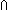x:AB(x) is the intersection of a family of types B(x) over x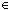A.

More precisely, u = v(x:AB(x)) when u = vB(x) for all xA (see Types). Consequently,

Thm*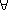B:(A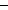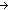Type), a:A. (x:AB(x))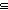B(a)

If A is empty, then (x:AB(x)) has exactly one member, and every (closed) expression denotes it (Thm* whatever1 = whatever2(x:Void. Whatever(x))). See Types.

The type (A:Type. (A List)(A List)), for example, is the type of "polymorphic" operations on lists that don't depend on the type of the elements. For example,

(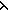as.rev(as))(A:Type. (A List)(A List))

where rev(as) is the list reversing function,

Def rev(as) == Case of as; nil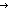nil ; a.as'rev(as') @ [a]  (recursive)

For more examples about intersection see Polymorphism.

Guarded Types: T(given A)

In the special case of "x:AB(x)" where some expression T not actually using x is used in place of B(x), then the intersection, denoted "T(given A)", is a way of "guarding" membership in T by whether A has a member.

If A has a member, then the type T(given A) is T (extensionally). If A is empty, then T(given A) is degenerate, having one member which is denoted by every closed expression (see Types).

So for example, in Nuprl, in order for AB to denote a type, A must also denote a type, but B need only denote a type if A has a member. If A is empty, then B may be any closed term, and AB then denotes simply the one-element function space with empty domain. See Function Types.

This well-formedness condition can be expressed using guarded types thus:

Thm*A:Type, B:Type(given A). ABType

The restriction of B to Type(given A) escapes the absolute condition of B simply being in Type.

See Guarded Types for more on guarded types and propositions.

(Oct 2001 - sfa )